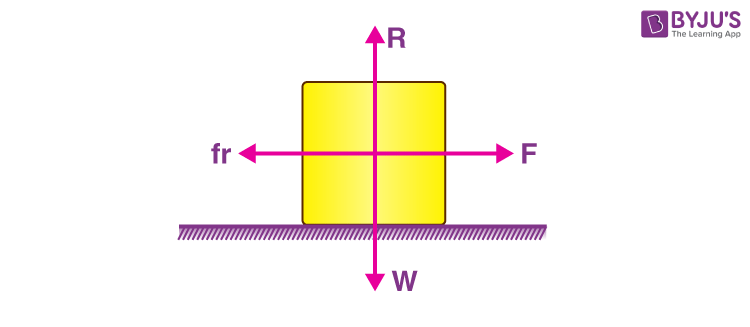# Static Friction

Friction can be defined as the force resisting the relative motion of fluid layers, solid surfaces and material elements sliding against each other. Friction can be classified into several categories:

• Fluid friction
• Lubricated friction
• Skin friction
• Internal friction
• Dry friction
• Kinetic friction
• Static friction

## What is Static Friction?

Static friction is a force that keeps an object at rest. Static friction definition can be written as:

The friction experienced when individuals try to move a stationary object on a surface, without actually triggering any relative motion between the body and the surface on which it is on.

It can be explained as the force of friction which precisely balances the applied force for the duration of the stationary state of the body.

The static frictional force is self-regulating, i.e. static friction will at all times be equivalent and opposite to the force applied.R is the reaction force because of the weight W. The external force is F, and fis the friction. F = -fr when no motion takes place.

## Watch the video to understand the nuances of static friction## Static Friction Examples

Some real-life examples of static friction are given in the points below.

• Papers on a tabletop
• A towel hanging on a rack
• A bookmark in a book
• A car parked on a hill

You may also want to check out the topics given below!

## Laws of Static Friction

• The maximum force of static friction is not dependent on the area of contact.
• The maximum force of static friction is comparative to the normal force i.e., if the normal force increases, the maximum external force that the object can endure without moving, also increases.

## Limiting Friction:

It is the highest value of static friction which comes into play when an object is just about to slide over the surface of a different object. For an exerted external force greater than the limiting friction, the body begins to move.

Once the motion has started, static friction cannot be considered. A new type of friction termed as kinetic friction comes into play.

### Laws of Limiting Friction:

• The direction of limiting frictional force is always contrary to the direction of motion.
• Limiting friction acts tangentially to the two surfaces interacting.
• The magnitude of limiting friction is proportional to the normal reaction between the two surfaces.
• The limiting friction hinges upon the material, the nature of the surfaces interacting and their evenness.
• So long as the normal reaction is the same, the magnitude of limiting friction is free of the shape or the area of the surfaces in contact for any two given surfaces.

## Watch the video and learn about friction, coefficient and properties of friction## Frequently Asked Questions – FAQs

Q1

### What is static friction?

The friction experienced when individuals try to move a stationary object on a surface, without actually triggering any relative motion between the body and the surface is known as static friction.
Q2

### What is sliding friction?

Sliding friction is defined as the resistance that is created between any two objects when they are sliding against each other.
Q3

### Is frictional force a scalar or a vector?

The frictional force is a vector since it involves both magnitude and direction.
Q4

### How does friction produce heat?

When surfaces in contact move relative to each other, the friction between the two surfaces converts kinetic energy into heat.
Q5

### What is the formula to calculate the frictional force?

The frictional force is given by the formula: F = μsN
Where μs is the coefficient of static friction and N is the normal reaction between the two contact surfaces.

## Understand the variable and self-adjusting nature of the static friction with the help of animationTest your Knowledge on Static Friction